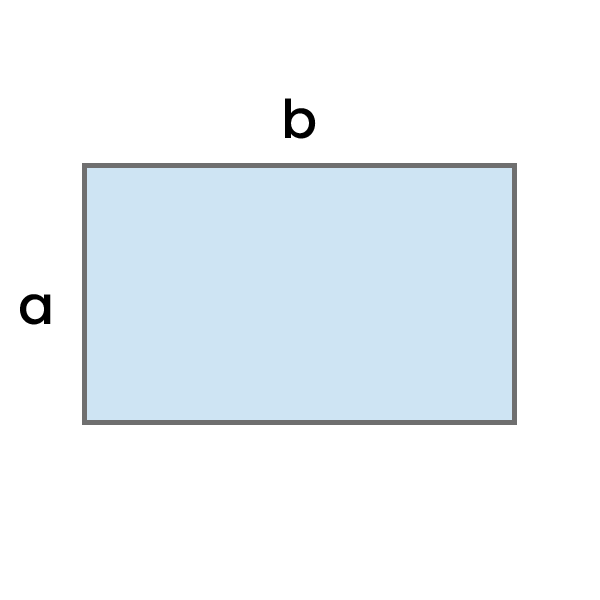# Area of a Rectangle Calculator

Enter the height (a) and width (b) of the rectangle in any units, and calculate its area. You can also see the detailed step-by-step calculation process.

Area = a * bResult:

## How to use this calculator?

1. Enter the height (a) and width (b) in the selected units.
2. See the calculated Area of a rectangle, and if needed, change its unit.
3. See the detailed, step-by-step Calculation process.

## How to calculate Area of a Rectangle?

To calculate the area of a rectangle, multiply the length of side "a" by the length of side "b."

Area of a Rectangle = a * b
• a - Height
• b - Width
Example: Calculate the area of a rectangle with height (a) of 6 cm and width (b) of 14 cm.

Area = a * b

Area = 6 cm * 14 cm

Area = 84 cm²